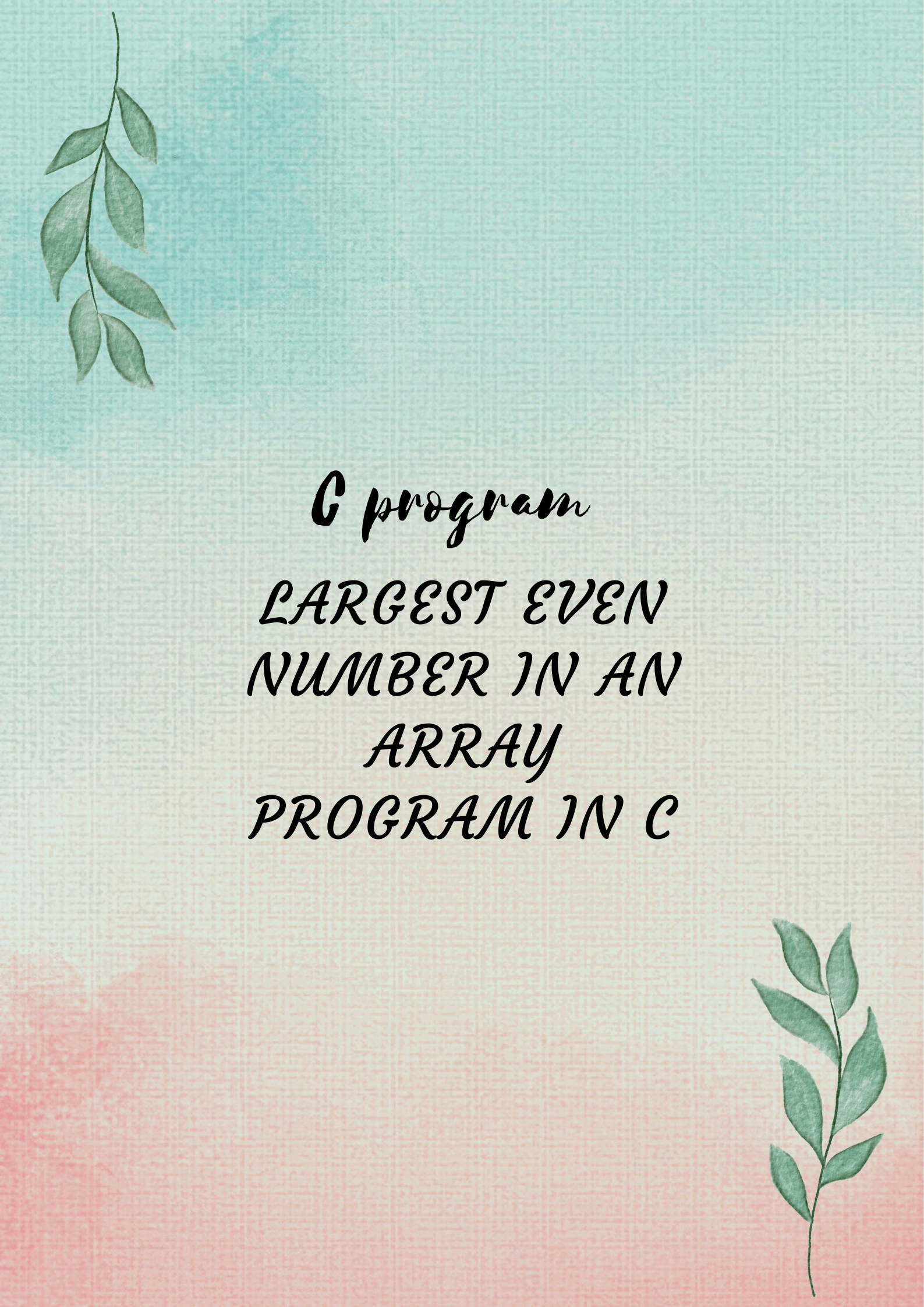September 25, 2022# Write a program to find the largest even number in an array

## Introduction of largest even number in an array

The task is to print the largest even number in an array. We have tried our best to explain the logic and program so we hope you find this implementation so go on and read.

Try to understand the implementation and it is easy to understand. There will be more upcoming articles to get more knowledge in C. Come back and read the article again if there is confusion.

Most importantly execute the program and try to get the correct output and if you follow the code, you will get it. Understanding the logic which is important, all the syntax errors are fine at first.

## Explanation of the logic

Similar to the largest number program, we considered the first index number to be the largest number and then compared it iteratively but for this program, if we consider the first index number in an array to be the largest then it might be an odd number and our logic will go wrong in this case. We do not want the odd number to be compared as our case is to find the largest even number in an array.

You can refer to the largest number program in an array by clicking on the below link.

The largest number Program in an array in C programming

## Logic to search the first even numbery=0;

While(y<=9)

{

if(x[y]%2==0)

{

break;

}

y++;

}

if(y==10)

{

Printf(“All are odd numbers”);

}

else

{

largestEven=x[y];

y++;

While(y<=9)

{

if(x[y]%2==0 && x[y]>largestEven)

{

largestEven=x[y];

}

y++;

}

Printf(“Largest even number is %d\n”,largest);

## Explanation of the largest even number in an array program

Note: With the help of the “break” keyword, control comes out of the loop.

The first while loop contains the if condition which determines the even number. If it is satisfied that it is an even number, the break keyword helps the control to come out of the while loop.

That even number is then stored in the largestEven variable and compared with the next number in an array.

This way the comparison continues and we get the output as the largest even number. But, if the condition of if does not satisfy then the control will go under the next if condition.

In that, if condition, it checks whether the index number is 10 or not. If it is 10 then the checking comes outside the array as y<=9. This concludes all the numbers are odd so we have printed that if y==10 satisfies.

## Accept 10 numbers and determine the largest even number.

`#include<stdio.h>`

`int main()`

`{`

int x,y,largestEven;

`y=0;`

`While(y<=9)`

`{`

`Printf("Enter a number");`

`Scanf("%d",&x[y]);`

`y++;`

`}`

`y=0;`

`While(y<=9)`

`{`

`if(x[y]%2==0)`

`{`

`break;`

`}`

`y++;`

`}`

`if(y==10)`

`{`

`Printf("All are odd numbers");`

`}`

`else`

`{`

`largestEven=x[y];`

`y++;`

`While(y<=9)`

`{`

`if(x[y]%2==0 && x[y]>largestEven)`

`{`

`largestEven=x[y];`

`}`

`y++;`

`}`

`Printf("The Largest even number is %d\n",largest);`

## Output

`Enter a number: 130`

`Enter a number: 15`

`Enter a number: 8`

`Enter a number: 70`

`Enter a number: 43`

`Enter a number: 59`

`Enter a number: 44`

`Enter a number: 33`

`Enter a number: 25`

`Enter a number: 18`

`The largest even number is 130`

`Enter a number: 130`

`Enter a number: 15`

`Enter a number: 9`

`Enter a number: 7`

`Enter a number: 43`

`Enter a number: 59`

`Enter a number: 43`

`Enter a number: 31`

`Enter a number: 27`

`Enter a number: 19`

`All are odd numbers`

## Conclusion of reverse the contents of an array program

The program is successful as you can see. Please try to write the program and run it. We have tried our best to explain the logic and program so we hope you find this implementation alternative and stay tuned for the upcoming blogs.

https://curledmark.in/the-largest-number-program-in-an-array-in-c-programming/

https://curledmark.in/armstrong-number-and-arrays-in-c-programming/

https://curledmark.in/prime-reversepower-programs-in-c-programming/

The Book Of Five Rings Summary

The Blue Umbrella

How to Talk to Anyone by Leil Lowndes

Jonathan Livingston Seagull Book Summary

Your Brain on PORN Book Summary by Gary Wilson

THE IMMORTALS OF MELUHA By Amish Tripathi full summary

Eleven Minutes by Paulo Coelho Summary

Hyperfocus by Chris Bailey Summary

Black Beauty by Anna Sewell Summary

You Can Heal Your Life by Louise Hay Summary

ATTITUDE IS EVERYTHING BY JEFF KELLER SUMMARY

THE BIOGRAPHY OF SWAMI VIVEKANANDA

THE SHAWSHANK REDEMPTION EXPLAINED

Turtles All the Way Down by John Green Story Explained

#### You may have missed#### What is do-while loop in C Programming ? Loops